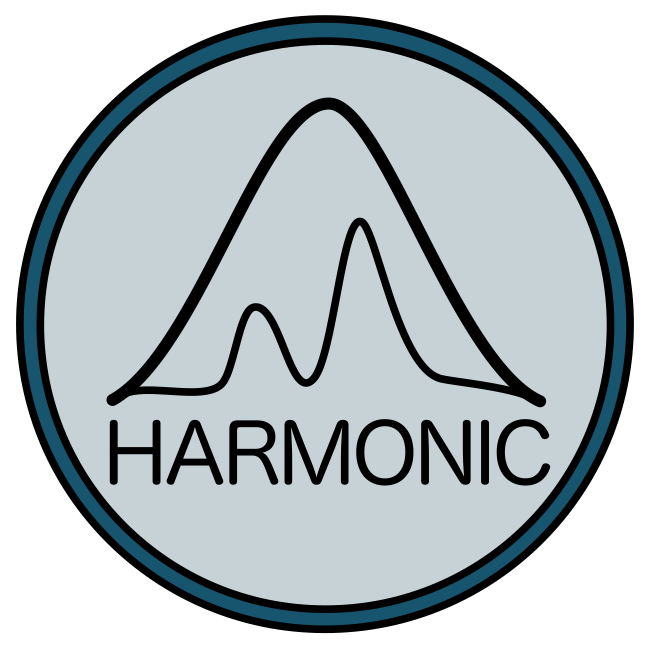# harmonic: Learnt harmonic mean estimator for Bayesian model selectionWe resurrect the infamous harmonic mean estimator for computing the marginal likelihood (Bayesian evidence) and solve its problematic large variance. The marginal likelihood is a key component of Bayesian model selection since it is required to evaluate model posterior probabilities; however, its computation is challenging. The original harmonic mean estimator, first proposed in 1994 by Newton and Raftery, involves computing the harmonic mean of the likelihood given samples from the posterior. It was immediately realised that the original estimator can fail catastrophically since its variance can become very large and may not be finite. A number of variants of the harmonic mean estimator have been proposed to address this issue although none have proven fully satisfactory.

We present the learnt harmonic mean estimator, a variant of the original estimator that solves its large variance problem. This is achieved by interpreting the harmonic me an estimator as importance sampling and introducing a new target distribution. The new target distribution is learned to approximate the optimal but inaccessible target, while minimising the variance of the resulting estimator. Since the estimator requires samples of the posterior only it is agnostic to the strategy used to generate posterior samples.